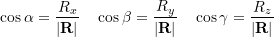### Vectors and their Operations: Vector operations using Cartesian vector notation

### Planar vector operations using CVN (two dimensions)

Addition of several vectors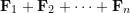using CVN takes the following steps:

1- Express each vector in CVN by resolving the vector to its scalar components: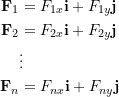2- Add the respective scalar components (components on the same axis):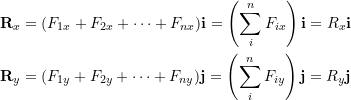in which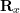and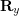are the Cartesian vector components of the resultant vector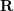.

The above steps can be summarized as:

(2.7)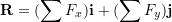in which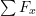and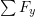represent the algebraic sums of the scalar components along theand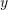axes respectively.

3- Form the resultant vector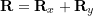. The magnitude ofand its direction with respect to theaxis can be obtained by,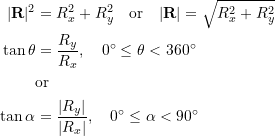Remark: the apparent location of a vector on a plane does not affect its CVN.

Planar vector addition using CVN is illustrated by the following interactive tool.

### Spatial vector Addition using CVN (three dimensions)

Once the vectors to be summed are resolved into their components and represented in CVN, the similar steps as in the coplanar case should be followed but with including components in thedirection. This means,

(2.8)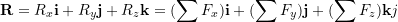in which,, and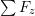represent the algebraic sums of the scalar components along the,andaxes respectively.

The magnitude of the resultant vector is,

(2.9)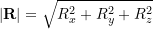The direction ofcan be expressed by the coordinate direction angles. The angles are determined using Eq 2.5 as,